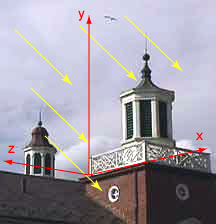Yes.

# Representing a VectorVectors are used for several purposes in 3D computer graphics. So they need to be represented in a way that programs can manipulate. And, just as you feared, this is done with numbers. The picture now includes our coordinate frame.

A vector is represented with a column matrix (as are points). It is somewhat confusing that both points and vectors are represented with the same thing. But this will prove to be convenient for computer manipulation.

To represent a vector as a column matrix:

1. Choose a coordinate frame.
2. Calculate the X length (positive or negative) from the tail of the vector to the tip.
3. Calculate the Y length (positive or negative) from the tail of the vector to the tip.
4. Calculate the Z length (positive or negative) from the tail of the vector to the tip.
5. Put those three numbers into a column.

A convenient way to do this is to position the tail of the vector at the origin, and then just read off the x, y, z values at the tip.

### QUESTION 16:

Try to do this with the picture.

1. For the arrows in the picture, how many feet in the X direction is the tip from the tail? ______
2. How many feet in the Y direction is the tip from the tail? ______
3. How many feet in the Z direction is the tip from the tail? ______